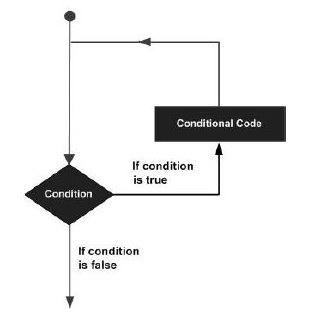# Rust - Loop

There may be instances, where a block of code needs to be executed repeatedly. In general, programming instructions are executed sequentially: The first statement in a function is executed first, followed by the second, and so on.

Programming languages provide various control structures that allow for more complicated execution paths.

A loop statement allows us to execute a statement or group of statements multiple times. Given below is the general form of a loop statement in most of the programming languages.Rust provides different types of loops to handle looping requirements −

• while
• loop
• for

## Definite Loop

A loop the number of iterations of which is definite/fixed is termed as a definite loop. The for loop is an implementation of a definite loop.

### For Loop

The for loop executes the code block for a specified number of times. It can be used to iterate over a fixed set of values, such as an array. The syntax of the for loop is as given below

### Syntax

```for temp_variable in lower_bound..upper_bound {
//statements
}
```

An example of a for loop is as shown below

```fn main(){
for x in 1..11{ // 11 is not inclusive
if x==5 {
continue;
}
println!("x is {}",x);
}
}
```

NOTE: that the variable x is only accessible within the for block.

### Output

```x is 1
x is 2
x is 3
x is 4
x is 6
x is 7
x is 8
x is 9
x is 10
```

## Indefinite Loop

An indefinite loop is used when the number of iterations in a loop is indeterminate or unknown.

Indefinite loops can be implemented using −

Sr.No Name & Description
1

While

The while loop executes the instructions each time the condition specified evaluates to true

2

Loop

The loop is a while(true) indefinite loop

### Illustration − for while

```fn main(){
let mut x = 0;
while x < 10{
x+=1;
println!("inside loop x value is {}",x);
}
println!("outside loop x value is {}",x);
}
```

The output is as shown below −

```inside loop x value is 1
inside loop x value is 2
inside loop x value is 3
inside loop x value is 4
inside loop x value is 5
inside loop x value is 6
inside loop x value is 7
inside loop x value is 8
inside loop x value is 9
inside loop x value is 10
outside loop x value is 10
```

### Illustration −loop

```fn main(){
//while true

let mut x = 0;
loop {
x+=1;
println!("x={}",x);

if x==15 {
break;
}
}
}
```

The break statement is used to take the control out of a construct. Using break in a loop causes the program to exit the loop.

```x=1
x=2
x=3
x=4
x=5
x=6
x=7
x=8
x=9
x=10
x=11
x=12
x=13
x=14
x=15
```

## Continue Statement

The continue statement skips the subsequent statements in the current iteration and takes the control back to the beginning of the loop. Unlike the break statement, the continue does not exit the loop. It terminates the current iteration and starts the subsequent iteration.

An example of the continue statement is given below.

```fn main() {

let mut count = 0;

for num in 0..21 {
if num % 2==0 {
continue;
}
count+=1;
}
println! (" The count of odd values between 0 and 20 is: {} ",count);
//outputs 10
}
```

The above example displays the number of even values between 0 and 20. The loop exits the current iteration if the number is even. This is achieved using the continue statement.

The count of odd values between 0 and 20 is 10